Next: Implementation issues Up: Theory Previous: A more robust smoothness

## Calculating the smoothness of Z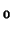The estimate of the covariance matrix for the residual field,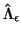, needs to be transformed to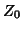for calculating the probabilities of clusters found in the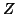-statistic image. The estimate of the covariance matrix for thefield,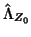can be computed by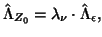(22)

where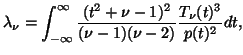(23)

where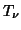is the PDF of a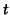-distribution with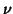degrees of freedom and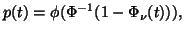(24)

where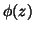is the PDF of the standard normal distribution.

David Flitney 2001-11-29